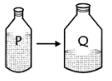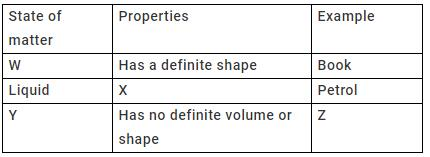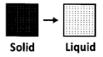Courses

# Test: Matter - 1

## 20 Questions MCQ Test Science Class 5 | Test: Matter - 1

Description
This mock test of Test: Matter - 1 for Class 5 helps you for every Class 5 entrance exam. This contains 20 Multiple Choice Questions for Class 5 Test: Matter - 1 (mcq) to study with solutions a complete question bank. The solved questions answers in this Test: Matter - 1 quiz give you a good mix of easy questions and tough questions. Class 5 students definitely take this Test: Matter - 1 exercise for a better result in the exam. You can find other Test: Matter - 1 extra questions, long questions & short questions for Class 5 on EduRev as well by searching above.
QUESTION: 1

### Matter can be defined as something that

Solution:

Matter is defined as something  that which occupies space and possesses rest mass, especially as distinct from energy and exists in all the three states.

QUESTION: 2

### Ice is the _______ form of water.

Solution:

Ice is the solid form of water.

QUESTION: 3

### The forces of attraction between molecules is greatest in the _________ state.

Solution:

In solids, the particles are tightly packed in a rigid pattern.

QUESTION: 4

The state of matter which has no fixed shape but has definite volume is the

Solution:

Matter in the liquid state has no fixed shape but has definite volume.

QUESTION: 5

Which of the following is true of the molecules of gases?

Solution:

The molecules of a gas move freely.

QUESTION: 6

Ice melts when energy in the form of________ is supplied.

Solution:

By the absorption of heat, ice melts to form water.

QUESTION: 7

In a bar of magnet the molecules are held by

Solution:

Bar magnet is a solid. In a solid, its molecules are held by strong forces of attraction.

QUESTION: 8

The process of changing water to water vapor is called

Solution:

When water is heated, it changes to water vapor. The process is called evaporation.

QUESTION: 9

A compound is formed when

Solution:

A compound is formed when two or more kinds of atoms combine.

QUESTION: 10

Matter is made up tiny particles called

Solution:

Matter is made up of tiny particles called atoms.

QUESTION: 11

Which of the following determines the state of matter?

Solution:

The state in which matter exists depends on how the molecules in it are packed.

QUESTION: 12

The intermolecular space between molecules is maximum in a

Solution:

In a gas, the molecules are far away from each other.

QUESTION: 13

When a solid is heated, it melts to form

Solution:

Solids melt to form liquids.

QUESTION: 14

Which of these processes involves change of state from the liquid to gaseous state?

Solution:

When water is heated, it evaporates to form water vapor.

QUESTION: 15

Substance 'P' does not have a fixed volume. It occupies the total space of the container and it can be compressed. What is 'P' likely to be?

Solution:

Gases have no strong bonds between the atoms. They can be compressed.

QUESTION: 16

Water present in container 'P' is transferred to container 'Q' as shown in the figure. Which of the following will undergo a change because of the transfer?Solution:

Liquids take the shape of the container, so the shape changes.

QUESTION: 17

When we heat a substance in solid form, it becomes liquid and then into gas. This shows that by heating, the space occupied by the substance generally:

Solution:

By heating the molecules moves apart, hence they occupy more space.

QUESTION: 18

In the table given below, states of matter, their properties and examples are represented by letters W, X, Y, Z.Q. Carbon dioxide can be placed in the place of letter________.

Solution:

Carbon dioxide is an example of gaseous state and so can be placed under z.

QUESTION: 19

The picture shows the process of _________.Solution:

The picture shows the process of melting.

QUESTION: 20

The force of attraction between molecules is the strongest in

Solution:

Figure A shows the strongest forces of attraction between the molecules of a solid.